# How to Sort Data but Keep Blank Cells in Excel

In daily work, if we sort data with blank cells included in the same column, these blank cells are listed at the bottom automatically after sorting. If we want to keep the positions of these blank cells unchanged and only sort the others, how can we do that? In this article, we will show you the way to sort data in this scenario.

## EXAMPLE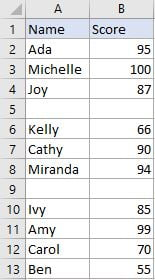->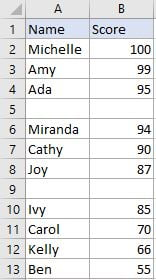There are two blank rows listed in above table. We want to sort data by score column with the two blank rows locked in row 5 and row 9. Normally, if we select score column and directly click sort “Z to A” button to sort data, the two blank cells are listed at the bottom, this behavior is not our expectation.

In fact, if we want to sort data with blank cells locked in their own positions, we can hide them firstly, then sort data in visible cells. After sorting data by proper order, we can unhide the hidden rows, the blank cells are still listed there.

## STEPS

a. Select range A2:B13, then click Home in the ribbon, in Editing section click Find & Select button to see options for finding texts in document.b. Click Go To Special option.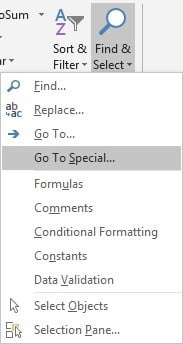c. In Go To Special dialog, check on “Blanks” option. Options in this dialog are exclusive. Then click OK button.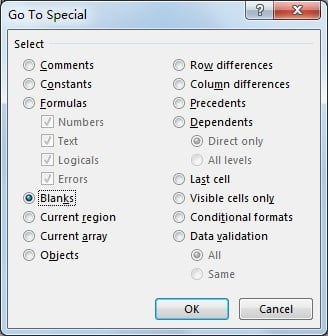d. After clicking OK, all blank cells are highlighted.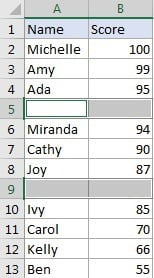e. Keep blank cells highlighted. Press Ctrl+9 to hide rows. In Excel, Ctrl+9 can hide rows with cell or range highlighted. If you want to unhide rows, you can press Ctrl+Shift+9. If you want to hide columns instead of rows, you can press hotkey Ctrl+0.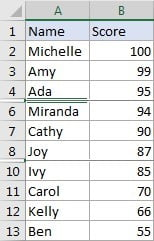f. Now, select B2:B13 with blank rows hidden, click Data in the ribbon, click Sort Largest to Smallest button in Sort & Filter section to sort numbers in B2:B13 from the largest to smallest.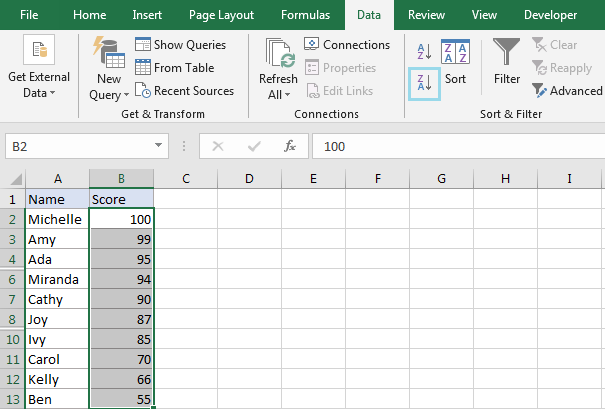g. After sorting, numbers are sorted from the largest to smallest properly.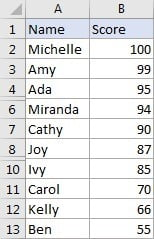h. Select entire rows from row 2 to row 13, right click to load row editing menu, click Unhide.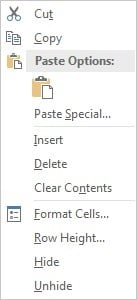Blank cells (rows) are still listed there.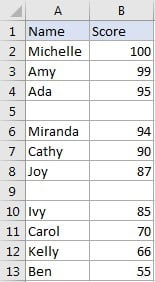Related Posts

Cap percentage values between 0 and 100

This article will talk about how to cap the percentage values between 0% and 100% in Microsoft Excel Spreadsheet or Google Sheets. If you are a newbie on Excel or google Sheets, you may be able to do this by ...

Calculate Cap Percentages to Specific Value

This article will talk about how to limit the cap percentage of a given amount to a given value in Microsoft Excel Spreadsheet or Google Sheets. If you are a newbie on Excel or google Sheets, you may be able ...

Calculate Win Loss Tie

Suppose you got a task to calculate the win, loss, and tie totals; what would you do? If you are new to Ms Excel and don't have enough experience with it, then you might do this task manually but let ...

Calculate Years Between Dates In Ms Excel

If you are an avid Ms Excel user, then you might have come across a task in which you needed to calculate the years between the dates; you might take it easy and do this task manually, which is also ...

Calculate Number of Hours between Two Times

Calculating the difference between two times might be a valuable statistic for subsequent computations or averages, whether you're producing a time sheet for staff or recording personal exercises. While Excel has a plethora of complex functions, including date and time ...

Calculate Loan Interest in Given Year

When you borrow money, you are supposed to repay it gradually. Lenders, on the other hand, want to be compensated for their services and the risk they incur by lending you money. That is, you will not just repay the ...

Calculate Interest Rate for Loan

The interest rate is the fee charged by a lender to a borrower and is expressed as a percentage of the principal—the lent amount. The interest rate on a loan is often expressed as an annual percentage rate, abbreviated as ...

Calculate Interest for Given Period

Using the IPMT function in Excel, we can compute the interest payment on any loan. This step-by-step tutorial will guide Excel users of all skill levels through the process to calculate interest for given period. Finally, the formula: =IPMT(B3/12,1,B5,-B2) The ...

How To Use Excel GCD Function

This post will guide you how to use Excel GCD function with syntax and examples in Microsoft excel. Description The Excel GCD function Returns the greatest common divisor of two or more integers. So you can use the GCD function ...

Calculate A Ratio From Two Numbers In Excel

In elementary mathematics, a ratio is a connection or comparison between two or more integers. For example, ratios are often expressed as ":" to demonstrate the relationship between two numbers. You would think that manually calculating a ratio from two ...

Sidebar